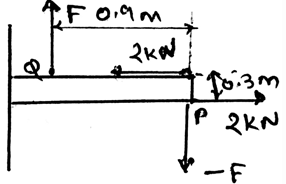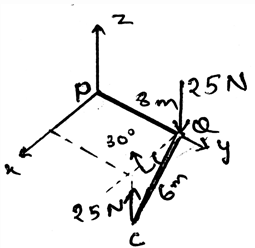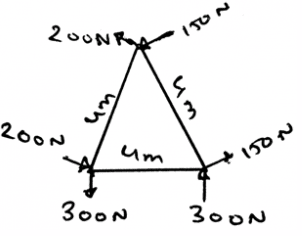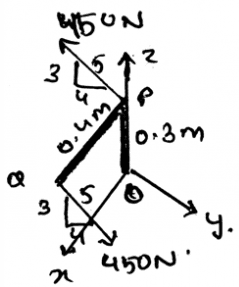Courses

# Test: Moment Of A Couple

## 15 Questions MCQ Test Engineering Mechanics | Test: Moment Of A Couple

Description
This mock test of Test: Moment Of A Couple for Mechanical Engineering helps you for every Mechanical Engineering entrance exam. This contains 15 Multiple Choice Questions for Mechanical Engineering Test: Moment Of A Couple (mcq) to study with solutions a complete question bank. The solved questions answers in this Test: Moment Of A Couple quiz give you a good mix of easy questions and tough questions. Mechanical Engineering students definitely take this Test: Moment Of A Couple exercise for a better result in the exam. You can find other Test: Moment Of A Couple extra questions, long questions & short questions for Mechanical Engineering on EduRev as well by searching above.
QUESTION: 1

### Couple is having a combination of two forces. They are different in magnitude.

Solution:

The couple is a combination of two forces of same magnitude. They are acting in the same sense of rotation. That is they are acting in the opposite direction, but giving the body a rotation in the same direction. Whether it may be in clockwise direction or anti-clockwise.

QUESTION: 2

Solution:
QUESTION: 3

### The distance between the forces acting in the couple is ____________

Solution:

The distance between the forces acting in the couple is having a finite distance. Couple is the combination of two forces which are acting in the same sense of rotation. That is they are acting in the opposite direction to provide a same direction rotation.

QUESTION: 4

The resultant force acting in the couple is _________

Solution:

The resultant of the force acting on the couple is always zero. As the force are acting in the opposite directions. So if we add the forces algebraically then the sum is going to be zero. Or in other words they cancel each other.

QUESTION: 5

A man is travelling in the car. He is driving the car. If he is taking a turn in the road. He is applying force to the steering wheel by holding the wheel with his both hands. The steering wheel is facing a moment of force.

Solution:

The man is trying to rotate the wheel by applying the force. The force is being applied on the steering wheel. Which is rotating the wheel, but it is the moment of the couple. Because the man has holded the wheel with his both hands, hence it is not the moment of the force.

QUESTION: 6

Which of the following is correct for the moment of couple M caused by two forces of same magnitude F and are separated by a distance of r?

Solution:

The answer is M = rxF. The moment id the cross product of the distance and the forces. It is the direction as directed by the curling of the hand. The thumb representing the direction of the moment. And the motion of the finger, that is the rotation gives the direction of the rotation caused by the forces.

QUESTION: 7

A couple moment is a _______ vector.

Solution:

A couple moment is a free vector. It can be act anywhere between the forces. As the couple is formed by two forces acting in the opposite directions. These forces are separated by a distance r, say, then the moment caused by them can act anywhere in between these forces.

QUESTION: 8

Determine the couple moment acting on the beam shown.Solution:

As we know that the moment is vector quantity, their summation requires vector math. The couple moment is also the same. That is they are also the vectors, and requires vector math. Thus the resultant couple moment is the vector sum of the various couples acting in the body.

QUESTION: 9

Which of the following is correct?

Solution:

The couple moment depends only on the distance vector between the forces. The radius vector of the forces are not making the couple depended over it. But the distance vector is driven by subtracting the radius vectors of the forces. This means that, moment of the couple doesn’t directly depends on the radius vector of forces.

QUESTION: 10

If the forces acting on the couple are in the same direction, that is they are not in the opposite direction as always they are, then?

Solution:

The forces are always in the opposite direction for the couple moment. But if they don’t act like the same, that is they change their directions and align themselves in the same direction then the couple is not possible.

QUESTION: 11

Determine the couple moment acting on the pipe shown.Solution:

As we know that the moment is vector quantity, their summation requires vector math. The couple moment is also the same. That is they are also the vectors, and requires vector math. Thus the resultant couple moment is the vector sum of the various couples acting in the body.

QUESTION: 12

The resultant couple moment is ____________ sum of various couples acting on the body.

Solution:

As we know that the moment is vector quantity, their summation requires vector math. The couple moment is also the same. That is they are also the vectors, and requires vector math. Thus the resultant couple moment is the vector sum of the various couples acting in the body.

QUESTION: 13

Determine the couple moment acting on the triangular plane shown.Solution:

As we know that the moment is vector quantity, their summation requires vector math. The couple moment is also the same. That is they are also the vectors, and requires vector math. Thus the resultant couple moment is the vector sum of the various couples acting in the body.

QUESTION: 14

If two forces magnitude F each are acting in the Cartesian coordinate and are acting at the points A(3,3) and B(-3,3). They are heading towards negative z direction and positive z direction respectively. The distance between them is given as r. The radius vectors of the force at A and B are Ra and Rb respectively. Then the moment is given as:

Solution:

The moment of couple as we know is the cross product of the force and the distance between them. But in this case we are given the radius vectors of the forces. So when we apply the vector maths to find the distance between the forces we get that the distance r = Rb-Ra. Now that we have the distance, we cross it with the force.

QUESTION: 15

Determine the couple moment acting on the pipe shown.Solution:

As we know that the moment is vector quantity, their summation requires vector math. The couple moment is also the same. That is they are also the vectors, and requires vector math. Thus the resultant couple moment is the vector sum of the various couples acting in the body.